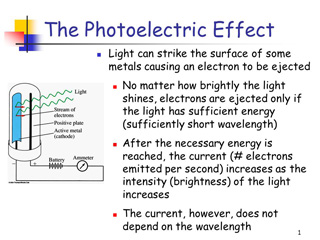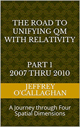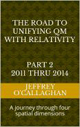# Particles as standing waves in space-time

It is possible, as this article will show that a standing wave in space-time is responsible for a photon.

A standing waves are created within a medium when the vibration frequency of the source causes reflected waves from one end of the medium to interfere with incident waves from the source. This interference occurs in such a manner that specific points along the medium appear to be standing still. Because the observed wave pattern is characterized by points that appear to be standing still, the pattern is often called a standing wave pattern. Such patterns are only created within the medium at specific frequencies of vibration. These frequencies are known as harmonic frequencies, or merely harmonics. At any frequency other than a harmonic frequency, the interference of reflected and incident waves leads to a resulting disturbance of the medium that is irregular and non-repeating.In March 1905 Einstein published a paper on the photoelectric effect entitled “On a Heuristic Viewpoint Concerning the Production and Transformation of Light” in which he proposed the idea of energy quanta and postulated light exists as tiny packets, or particles, called photons.

In that paper he stated Energy, in the propagation of a ray of light, is not continuously distributed over steadily increasing spaces, but it consists of a finite number of energy quanta but he did not say why.

Even so many fell the idea of light quanta contradicts the wave theory of light that follows naturally from James Clerk Maxwell’s equations for electromagnetic behavior and, more generally, the assumption of infinite divisibility of energy in physical systems.

Additionally he did not address the issue of how these “tiny packets” of energy called photons can move through space at the speed of light. This present a problem because he showed that energy and mass are equivalent and that the mass of any object or particle moving at the speed of is infinite.
Therefore, if energy is equivalent to mass one would assume that energy required to move a photon at the speed of light would be infinite.

However, Einstein gave us a way to define electromagnetic energy in a manner which is not only consistent with his theories but also with our classical understanding of nature when, in his General Theory of Relativity he showed that matter can be converted into energy or energy into matter according to the equation E=mc2.

For example Einstein defined the origin of the mass component of particles and all other objects, such as the sun in terms of curvature or distortion in the continuous field properties of space-time not in terms of their particle properties.

QED defines the fundamental unit (quanta) of light as “bundles of pure energy traveling at the speed of light with the unique property of being both particle and wave. However this means that as light moves through space-time the peaks and troughs of its wave properties would cause positive and negative spatial displacements in the “surface’ of space time.

Yet, it is difficult to understand how a spatial displacement can be responsible for of electromagnetism and how and why its wave properties morph to the particle QED defines as the photon when it is observed or interacts with matter because he CHOSE to use time or a displacement in space-time dimension to define mass and energy and not its spatial properties.

Yet he gave us the ability to form a physical image of this how the spatial properties of a photon’s wave packet are responsible for its movement through space when he defined its geometric properties in terms of the constant velocity of light and a dynamic balance between mass and energy because that provided a method of converting a unit of time in a space-time environment to a unit of space in four *spatial* dimensions. Additionally because the velocity of light is constant he also defined a one to one quantitative and qualitative correspondence between his space-time universe and one made up of four *spatial* dimensions.

The fact that one can use Einstein’s equations to qualitatively and quantitatively redefine the curvature in space-time he associated with energy in terms of four *spatial* dimensions is one bases for assuming as was done in the article ‘Defining energy?â’ Nov 27, 2007 that all forms of matter and energy can be derived in terms of a spatial displacement in a ‘surface’ of a three-dimensional space manifold with respect to a fourth *spatial* dimension.

However, this change in perspective gives one the ability to understand how the energy of a photon can move through space at the speed of light why it becomes a particle when interacting with matter in terms of the concepts of his theories.

For example as waves travel through water; they do not take the water with them because as wave arrives it lifts the water particles, they then travel forward, down and back so that each particle completes a circle. Circling movements of particles near the surface set off smaller circling movements below them therefore the waves don’t actually move the water forward. In other words the particles in a wave do not move with respect to space but exchange their potential energy of the water for kinetic energy associated with the wave’s movement.

Similar to wave on water the trough of a light wave would create a point with a positive curvature on a “surface” of the three-dimensional space manifold with respect to a fourth *spatial dimension which would present itself as the potential energy Einstein associated with mass. That point in space would then travel forward and up and back so that each one completed a circle without moving with respect to background of space. As the wave passed this point the potential energy of positive curvature in four “spatial” dimensions Einstein associated with mass would be converted to kinetic energy associated with a moving mass. In other words the wave packet of a photon can move though space at the speed of light because similar to a wave on water light waves do not cause a point in space to move with respect to the background of space-time.

This suggest that light is not electromagnetic wave but an energy wave in space-time which is the result of the potential energy created by the trough of a wave on its “surface” being converted to the kinetic energy associated with its peak thereby causing what is called light to move through space.

However, this also tell us when viewed in terms of their spatial properties that the electromagnetic properties of a light wave are the result of its propagation and not the casualty as is suggested by Maxwell’s equations.

(Later it will be shown in terms of those spatial properties the reason why this wave becomes a particle when interacting with matter but for now we would like to focus our attention on electromagnetic properties of light or a photon’s wave packet)

As was mention earlier a wave on the two-dimensional surface of water causes a point on that surface to be become displaced or rise above or below the equilibrium point that existed before the wave was present. A force will be developed by the differential displacement of the surfaces, which will result in the elevated and depressed portions of the water moving towards or become “attracted” to each other and the surface of the water.

Similarly a matter wave on the “surface” of a three-dimensional space manifold with respect to a fourth *spatial* dimension would cause a point on that “surface” to become displaced or rise above and below the equilibrium point that existed before the wave was present.

Therefore, classical wave mechanics, if extrapolated to four *spatial* dimensions tells us a force will be developed by the differential displacements caused by a matter wave moving on a “surface” of three-dimensional space with respect to a fourth *spatial* dimension that will result in its elevated and depressed portions moving towards or become “attracted” to each other.

This defines the causality of the attractive forces of unlike charges associated with the electromagnetic wave component of a photon in terms of a force developed by a differential displacement of a point on a “surface” of a three-dimensional space manifold with respect to a fourth *spatial* dimension.

However, it also provides a classical mechanism for understanding why similar charges repel each other because observations of water show that there is a direct relationship between the magnitudes of a displacement in its surface to the magnitude of the force resisting that displacement.

Similarly the magnitude of a displacement in a “surface” of a three-dimensional space manifold with respect to a fourth *spatial* dimension caused by two similar charges will be greater than that caused by a single one. Therefore, similar charges will repel each other because the magnitude of the force resisting the displacement will be greater for two charges than it would be for a single charge.

One can define the causality of electrical component of electromagnetic radiation in terms of the energy associated with its “peaks” and “troughs” that is directed perpendicular to its velocity vector while its magnetic component would be associated with the horizontal force developed by that perpendicular displacement.

However, Classical Mechanics tells us a horizontal force will be developed by that perpendicular or vertical displacement which will always be 90 degrees out of phase with it. This force is called magnetism.

This is analogous to how the vertical force pushing up of on mountain also generates a horizontal force, which pulls matter horizontally towards the apex of that displacement.

This shows that one can use the spatial properties of Einstein’s theories to derive causality of the electromagnetic forces of light and how the wave packet Quantum Electrodynamics associates with a photon is propagated through space by extrapolating the laws of classical mechanics in a three-dimensional environment to one consisting of four dimensional space-time or four *spatial* dimensions.

However viewing a light in terms of the spatial instead of the time properties of his theories allows one to understand how why it always appears as a particle when measured or observed.

For example in the article ‘Why is energy/mass quantized?’ Oct. 4, 2007 it was shown one can physical derive photonic properties of light by extrapolating the laws of classical wave mechanics in a three-dimensional environment to a matter energy wave on a “surface” of a three-dimensional space manifold with respect to a fourth *spatial* dimension.

Briefly it showed the four conditions required for resonance to occur in a classical environment, an object, or substance with a natural frequency, a forcing function at the same frequency as the natural frequency, the lack of a damping frequency and the ability for the substance to oscillate spatial would occur in one consisting of four spatial dimensions.

The existence of four *spatial* dimensions would give a matter wave the ability to oscillate spatially on a “surface” between a third and fourth *spatial* dimensions thereby fulfilling one of the requirements for classical resonance to occur.

These oscillations would be caused by an event such as the decay of a subatomic particle or the shifting of an electron in an atomic orbital. This would force the “surface” of a three-dimensional space manifold to oscillate with the frequency associated with the energy of that event.

The oscillations caused by such an event would serve as forcing function allowing a resonant system or “structure” to be established space.

Therefore, these oscillations in a “surface” of a three-dimensional space manifold would meet the requirements mentioned above for the formation of a resonant system or “structure” in four-dimensional space if one extrapolated them to that environment.

Classical mechanics tells us the energy of a resonant system can only take on the discrete or quantized values associated with it fundamental or a harmonic of its fundamental frequency.

Hence, these resonant systems in four *spatial* dimensions would be responsible for the discrete quantized energy associated with the quantum mechanical properties of a photon.

Yet one can also define the boundary conditions required to establish the standing wave component of a resonant system mentioned earlier that is responsible for it its particle properties as defined by QED.

For example in classical physics, a point on the two-dimensional surface of paper is confined to that surface. However, that surface can oscillate up or down with respect to three-dimensional space.

Similarly an object occupying a volume of three-dimensional space would be confined to it however, it could, similar to the surface of the paper oscillate “up” or “down” with respect to a fourth *spatial* dimension.

The confinement of the “upward” and “downward” oscillations of the field properties of mass with respect to a fourth *spatial* dimension is what defines the spatial boundaries that enables the formation of a resonant system which the article  “Why is energy/mass quantized?” defined as being responsible for a particle.

When a wave on water comes ashore the energy associated with its wave properties is confined to a specific region of the shoreline.

Similarly when a photon’s wave packet is measured or observed a portion of its wave energy is transmitted to the measuring instrument while some of it may be redirected or reflected similar to a wave striking the shoreline. In other word the energy wave which earlier was define as being responsible for the transmission of light interacts with measuring equipment for the same reason a water wave interacts with the shoreline.

However it also explains why light is always observed as a particle when it encounters encounters a measuring instrument or is observed.

In the quantum mechanical system described above such as light interacting with a particle, resonance only occurs when the frequency at which the force applied is equal or nearly equal to one or a multiple of the natural frequencies of the system on which it acts. In other words light when confined to three-dimensional space by interaction with a particle it will always present itself as the resonant structure that has the energy equal to one or a multiple of the natural frequency of space time. The remaining energy will be radiated through space as light with a lower frequency.

In other words the particle component of light or an electromagnetic wave is not the cause of its interaction with particles but a result of it.

However one can use the above model to explain why photons do not interact with each other because similar to waves on water if their is no obstruction to hinder their movement a wave will not interact with each other.  In other words, photon do not interfere with each other for the same reason that all energy waves do not.

Summing up, Einstein genius allows us to view his theory in either four dimensional space-time or four spatial dimensions. As was shown above changing ones perspective on his theory from time to its spatial equivalent allows one to define light as an energy wave in space and shows the electromagnetic properties are the result NOT the casualty of its propagation but a result of it. Similarly it shows the particle component of light is not the cause of its interaction with a particle but a result of it.It is important to note the validity these conclusions cannot be falsified if one accepts the validity of his theories.

Later Jeff

 The Road to Unifying QM with Relativity part 1 2007 thru 2010Ebook \$8.00Paper Back \$15.00 The Road to Unifying QM with Relativity part 2 2011 thru 2014Ebook \$8.00Paper Back \$16.00 The Road to Unifying QM with Relativity part 3 2015 thru 2020Ebook \$8.00Paper Back \$18.00